Скачать презентацию The Goods Market Lecture 17 academic year

4a4e560cea035da632d3d8a1c099a9cf.ppt

• Количество слайдов: 29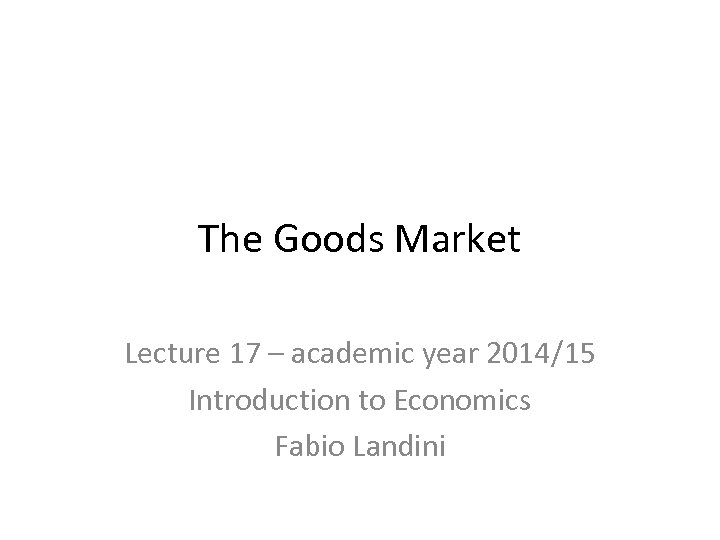The Goods Market Lecture 17 – academic year 2014/15 Introduction to Economics Fabio Landini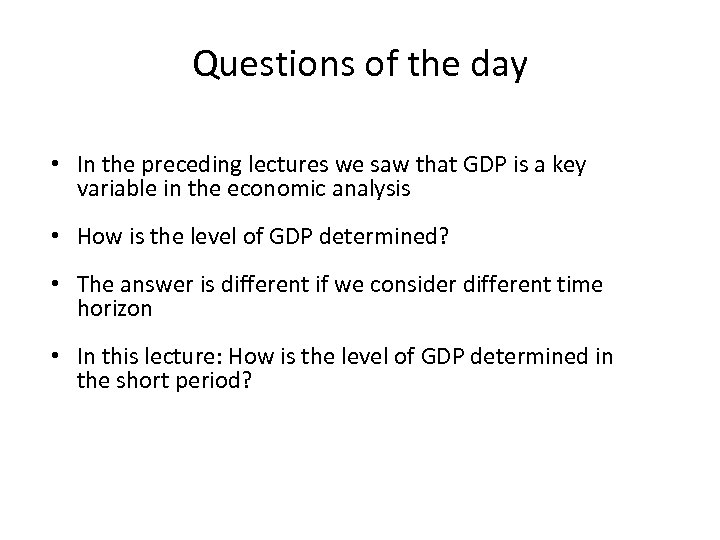Questions of the day • In the preceding lectures we saw that GDP is a key variable in the economic analysis • How is the level of GDP determined? • The answer is different if we consider different time horizon • In this lecture: How is the level of GDP determined in the short period?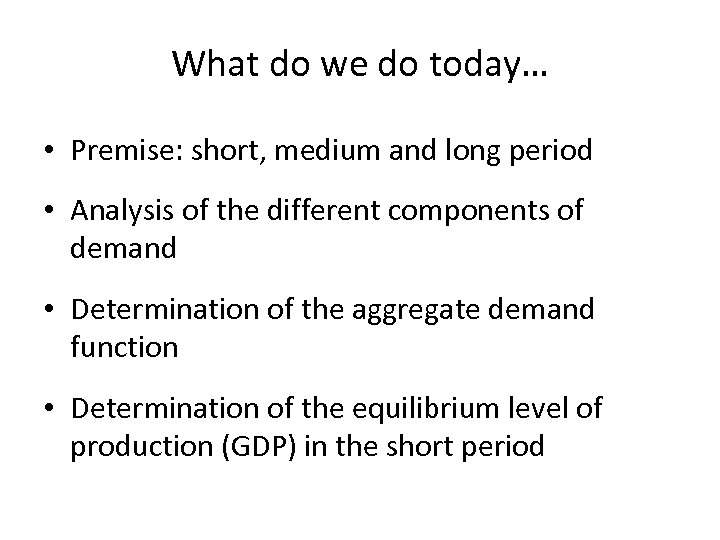What do we do today… • Premise: short, medium and long period • Analysis of the different components of demand • Determination of the aggregate demand function • Determination of the equilibrium level of production (GDP) in the short period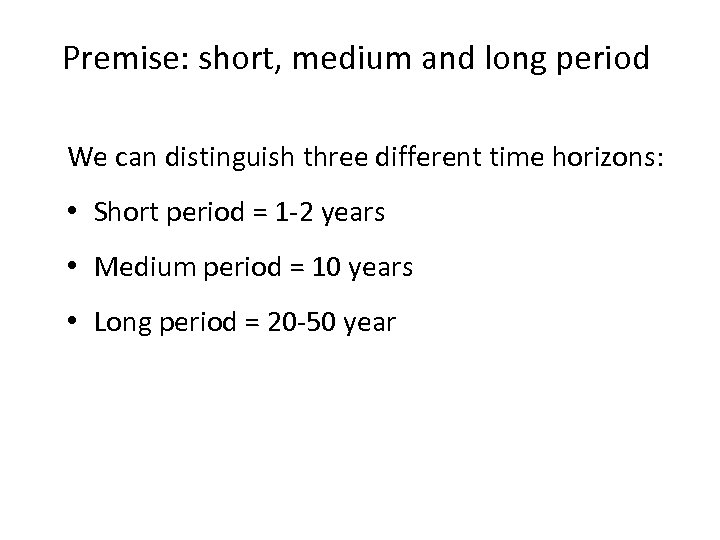Premise: short, medium and long period We can distinguish three different time horizons: • Short period = 1 -2 years • Medium period = 10 years • Long period = 20 -50 year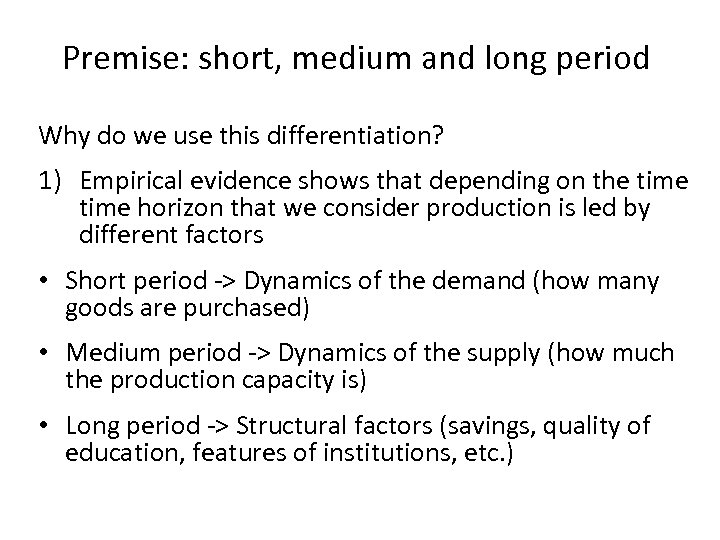Premise: short, medium and long period Why do we use this differentiation? 1) Empirical evidence shows that depending on the time horizon that we consider production is led by different factors • Short period -> Dynamics of the demand (how many goods are purchased) • Medium period -> Dynamics of the supply (how much the production capacity is) • Long period -> Structural factors (savings, quality of education, features of institutions, etc. )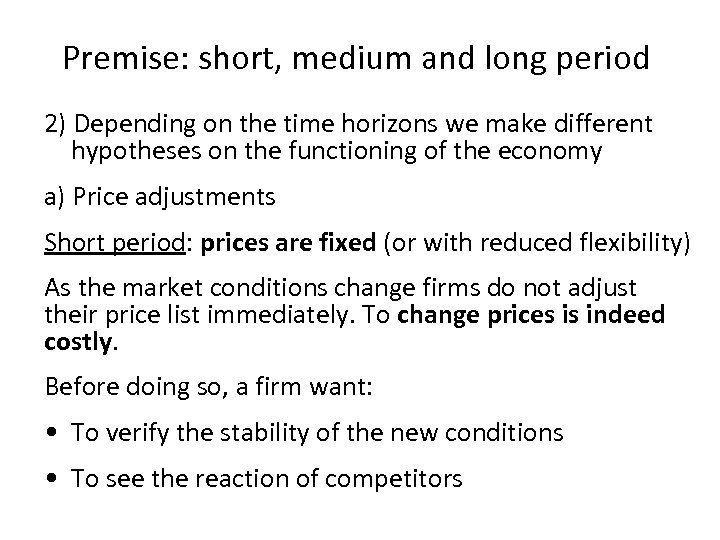Premise: short, medium and long period 2) Depending on the time horizons we make different hypotheses on the functioning of the economy a) Price adjustments Short period: prices are fixed (or with reduced flexibility) As the market conditions change firms do not adjust their price list immediately. To change prices is indeed costly. Before doing so, a firm want: • To verify the stability of the new conditions • To see the reaction of competitors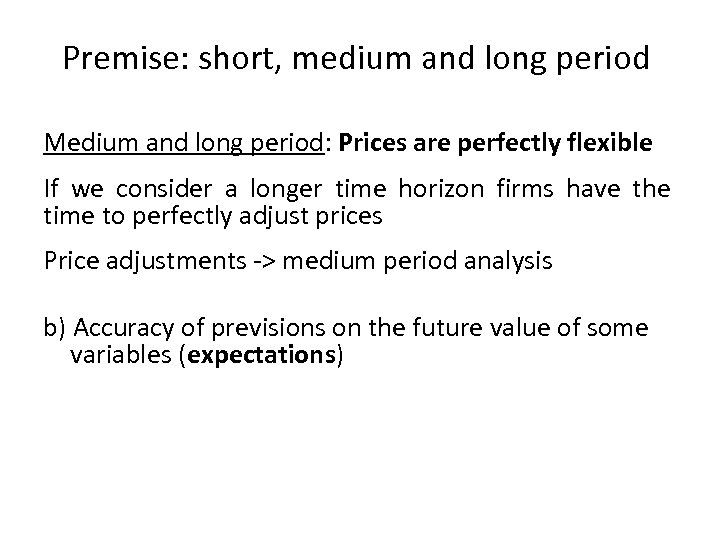Premise: short, medium and long period Medium and long period: Prices are perfectly flexible If we consider a longer time horizon firms have the time to perfectly adjust prices Price adjustments -> medium period analysis b) Accuracy of previsions on the future value of some variables (expectations)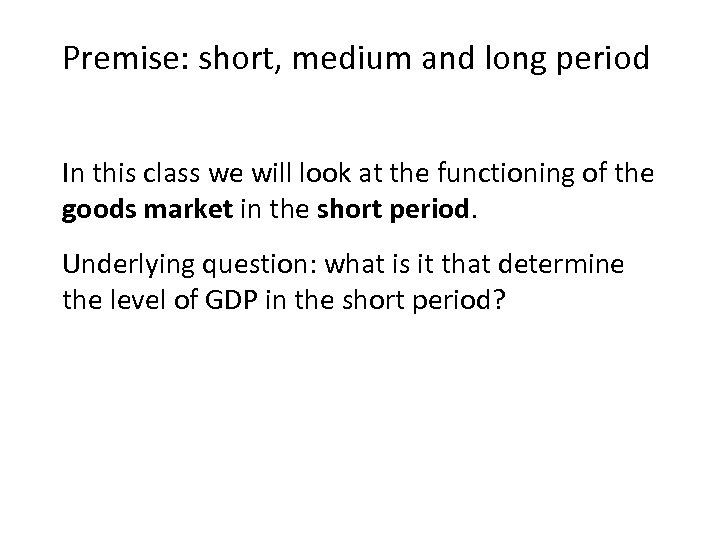Premise: short, medium and long period In this class we will look at the functioning of the goods market in the short period. Underlying question: what is it that determine the level of GDP in the short period?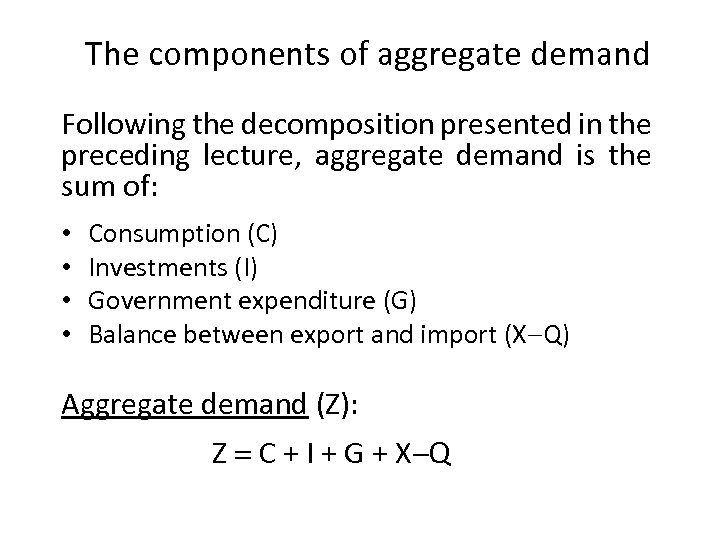The components of aggregate demand Following the decomposition presented in the preceding lecture, aggregate demand is the sum of: • • Consumption (C) Investments (I) Government expenditure (G) Balance between export and import (X-Q) Aggregate demand (Z): Z = C + I + G + X-Q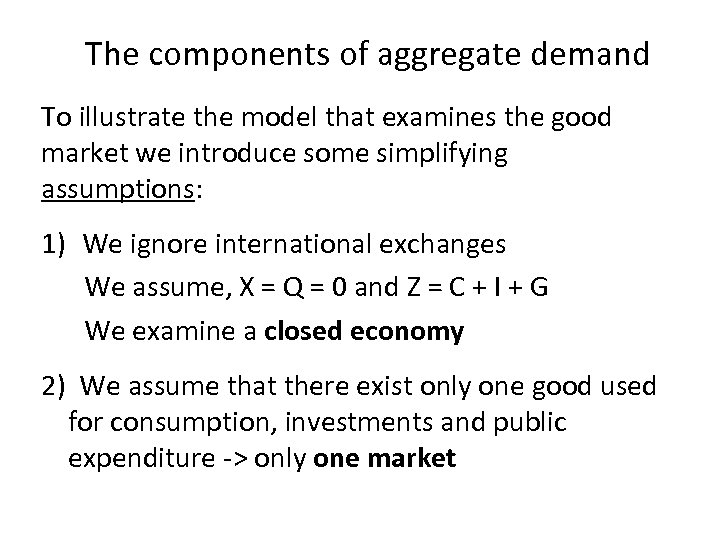The components of aggregate demand To illustrate the model that examines the good market we introduce some simplifying assumptions: 1) We ignore international exchanges We assume, X = Q = 0 and Z = C + I + G We examine a closed economy 2) We assume that there exist only one good used for consumption, investments and public expenditure -> only one market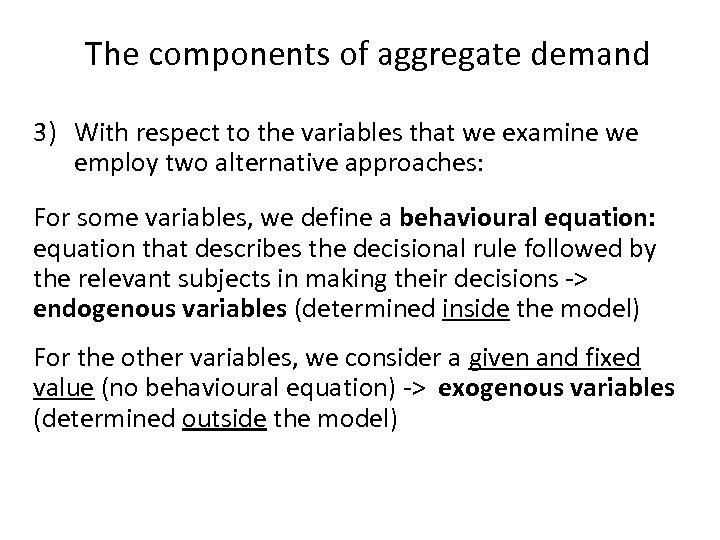The components of aggregate demand 3) With respect to the variables that we examine we employ two alternative approaches: For some variables, we define a behavioural equation: equation that describes the decisional rule followed by the relevant subjects in making their decisions -> endogenous variables (determined inside the model) For the other variables, we consider a given and fixed value (no behavioural equation) -> exogenous variables (determined outside the model)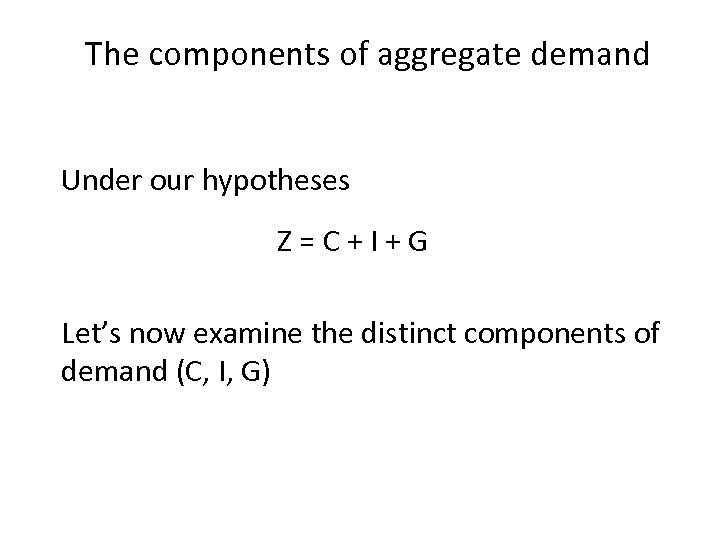The components of aggregate demand Under our hypotheses Z=C+I+G Let’s now examine the distinct components of demand (C, I, G)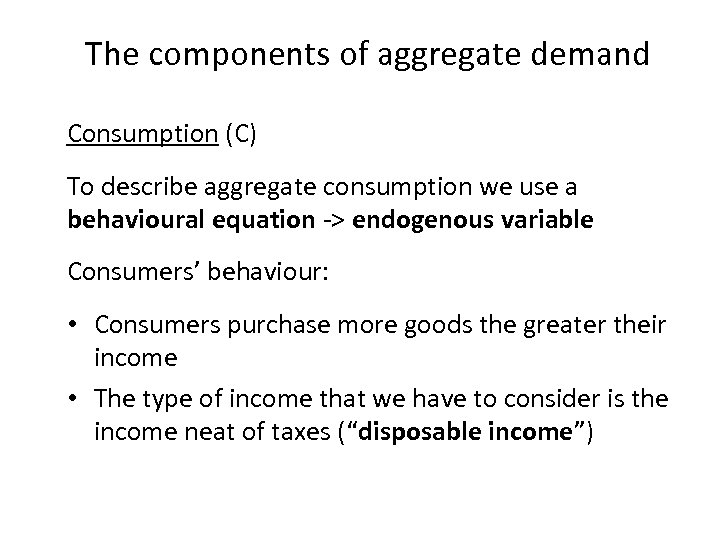The components of aggregate demand Consumption (C) To describe aggregate consumption we use a behavioural equation -> endogenous variable Consumers’ behaviour: • Consumers purchase more goods the greater their income • The type of income that we have to consider is the income neat of taxes (“disposable income”)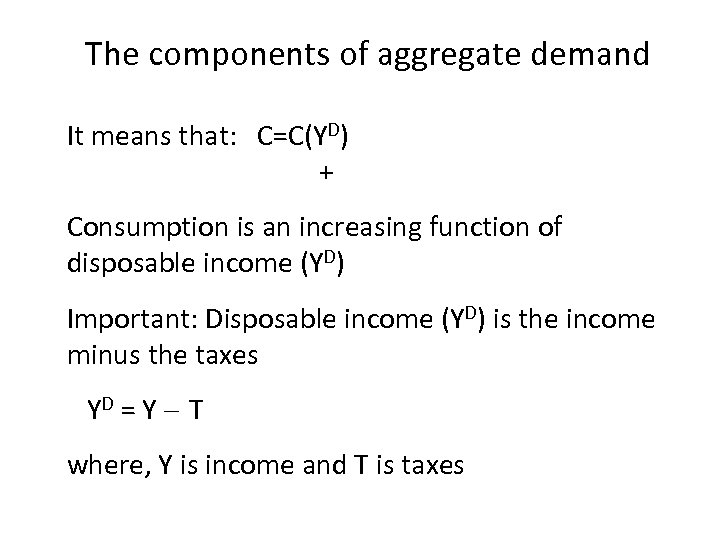The components of aggregate demand It means that: C=C(YD) + Consumption is an increasing function of disposable income (YD) Important: Disposable income (YD) is the income minus the taxes YD = Y – T where, Y is income and T is taxes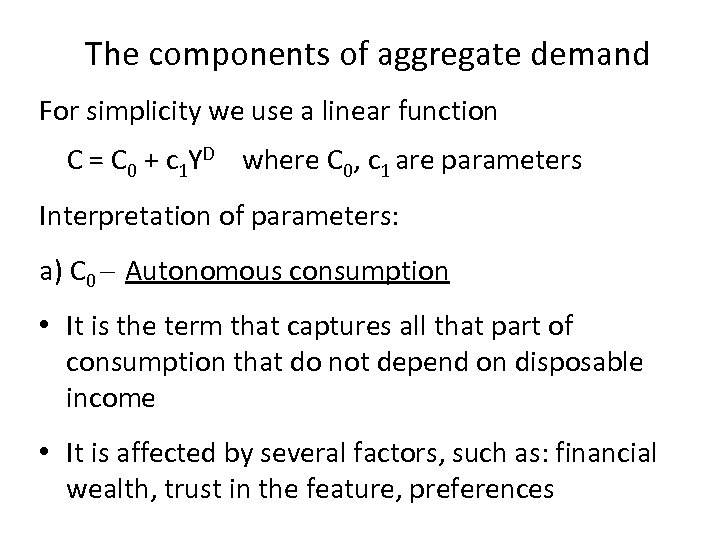The components of aggregate demand For simplicity we use a linear function C = C 0 + c 1 YD where C 0, c 1 are parameters Interpretation of parameters: a) C 0 – Autonomous consumption • It is the term that captures all that part of consumption that do not depend on disposable income • It is affected by several factors, such as: financial wealth, trust in the feature, preferences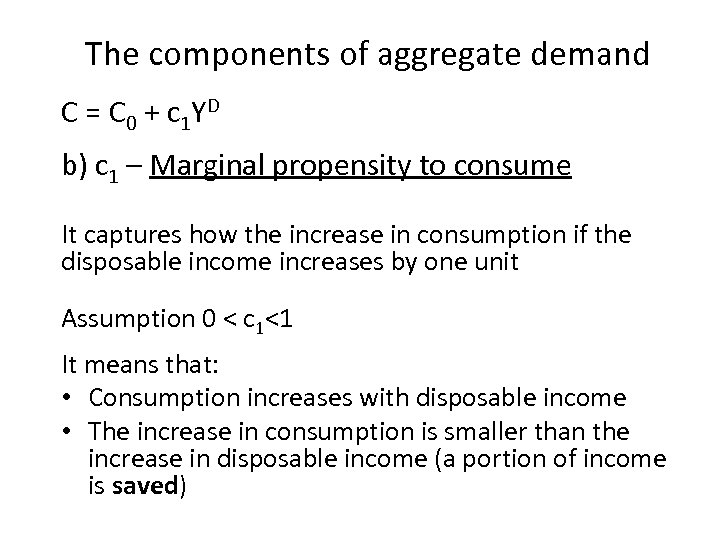The components of aggregate demand C = C 0 + c 1 YD b) c 1 – Marginal propensity to consume It captures how the increase in consumption if the disposable income increases by one unit Assumption 0 < c 1<1 It means that: • Consumption increases with disposable income • The increase in consumption is smaller than the increase in disposable income (a portion of income is saved)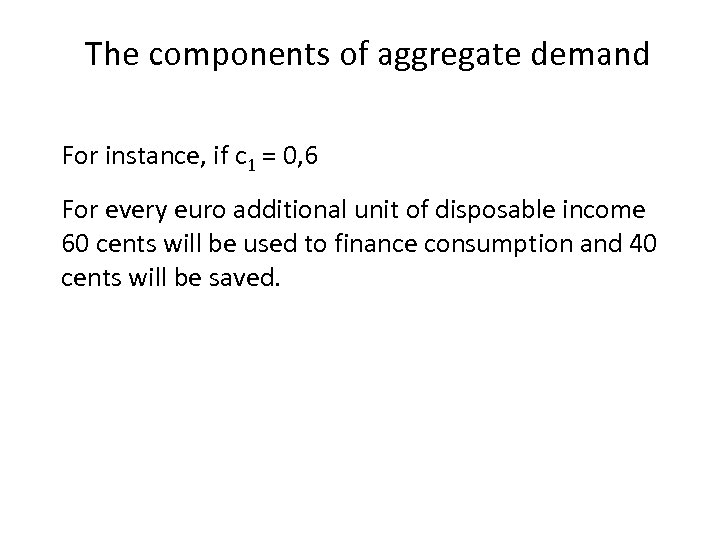The components of aggregate demand For instance, if c 1 = 0, 6 For every euro additional unit of disposable income 60 cents will be used to finance consumption and 40 cents will be saved.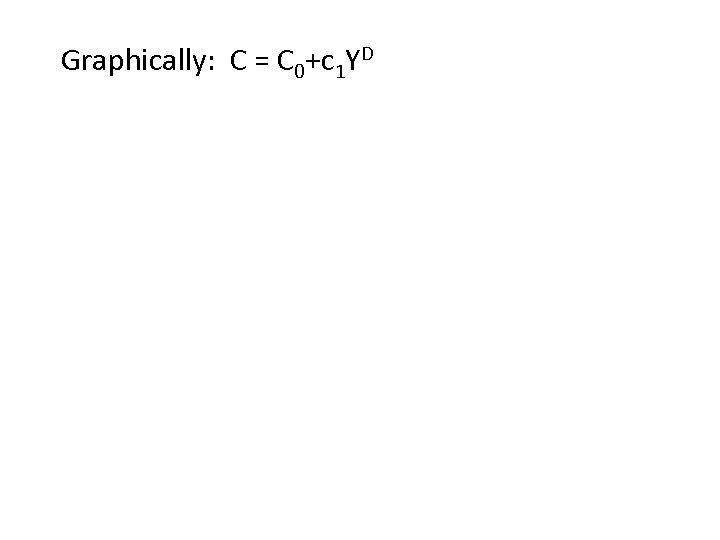Graphically: C = C 0+c 1 YD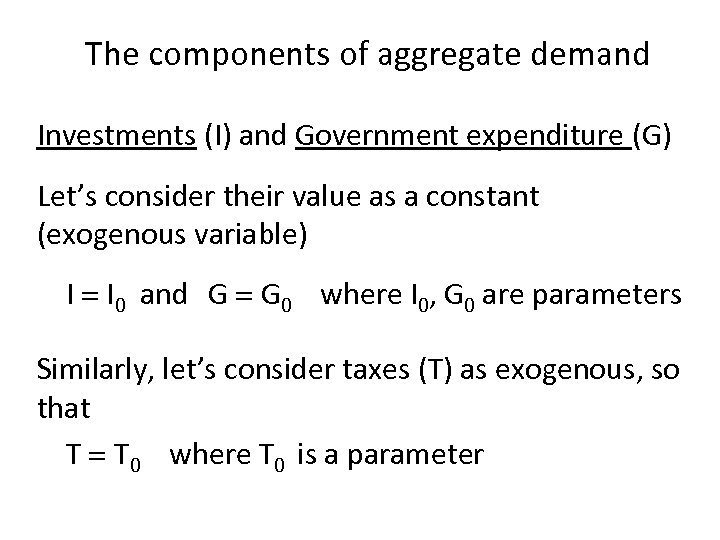The components of aggregate demand Investments (I) and Government expenditure (G) Let’s consider their value as a constant (exogenous variable) I = I 0 and G = G 0 where I 0, G 0 are parameters Similarly, let’s consider taxes (T) as exogenous, so that T = T 0 where T 0 is a parameter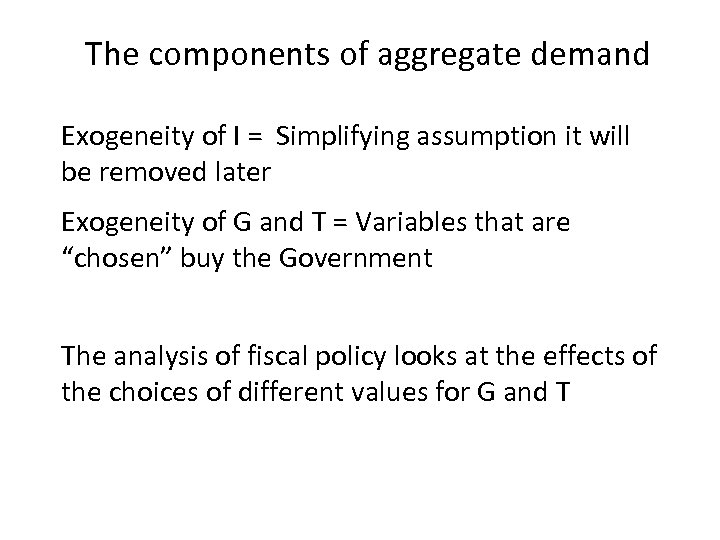The components of aggregate demand Exogeneity of I = Simplifying assumption it will be removed later Exogeneity of G and T = Variables that are “chosen” buy the Government The analysis of fiscal policy looks at the effects of the choices of different values for G and T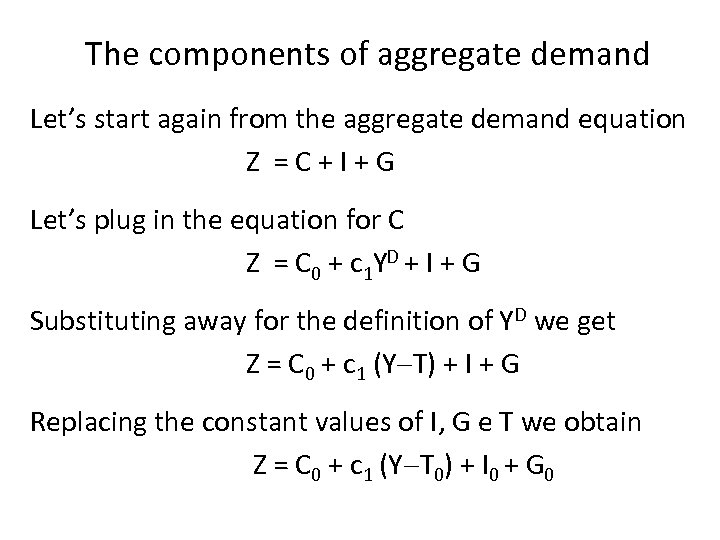The components of aggregate demand Let’s start again from the aggregate demand equation Z =C+I+G Let’s plug in the equation for C Z = C 0 + c 1 YD + I + G Substituting away for the definition of YD we get Z = C 0 + c 1 (Y-T) + I + G Replacing the constant values of I, G e T we obtain Z = C 0 + c 1 (Y-T 0) + I 0 + G 0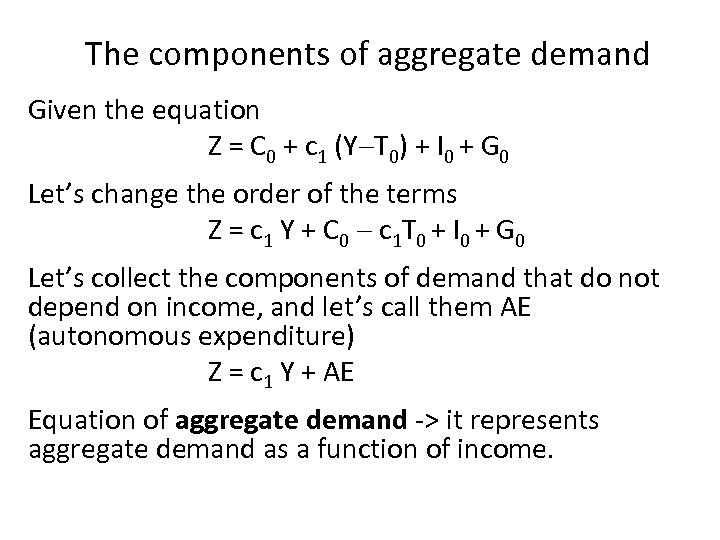The components of aggregate demand Given the equation Z = C 0 + c 1 (Y-T 0) + I 0 + G 0 Let’s change the order of the terms Z = c 1 Y + C 0 - c 1 T 0 + I 0 + G 0 Let’s collect the components of demand that do not depend on income, and let’s call them AE (autonomous expenditure) Z = c 1 Y + AE Equation of aggregate demand -> it represents aggregate demand as a function of income.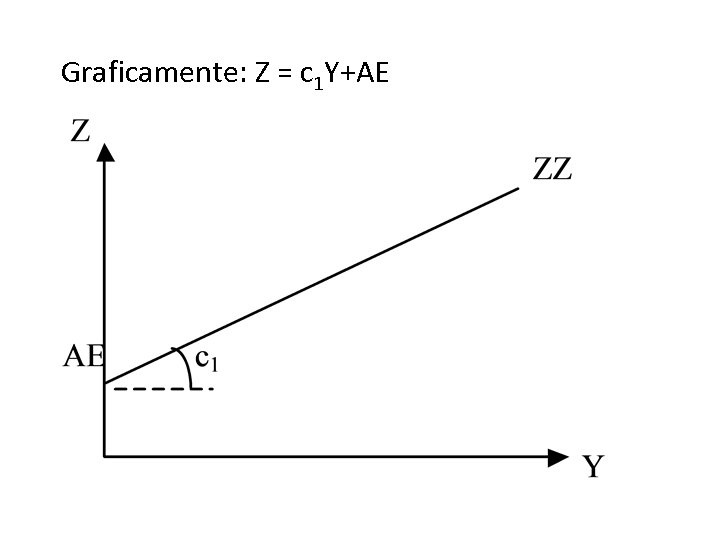Graficamente: Z = c 1 Y+AE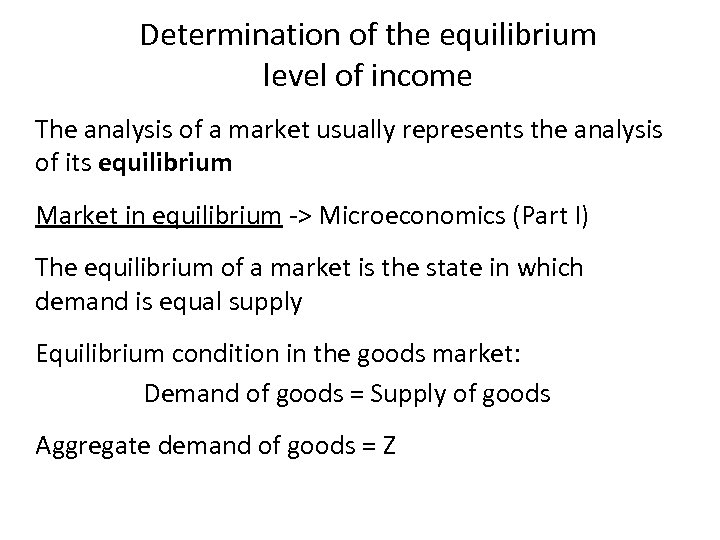Determination of the equilibrium level of income The analysis of a market usually represents the analysis of its equilibrium Market in equilibrium -> Microeconomics (Part I) The equilibrium of a market is the state in which demand is equal supply Equilibrium condition in the goods market: Demand of goods = Supply of goods Aggregate demand of goods = Z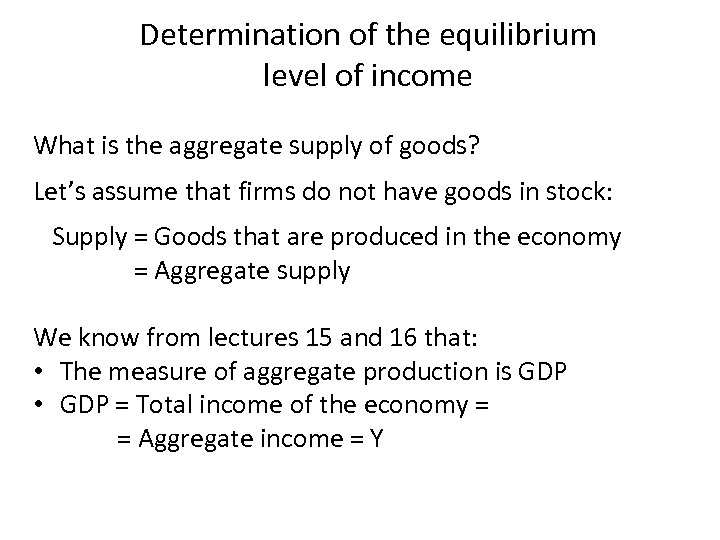Determination of the equilibrium level of income What is the aggregate supply of goods? Let’s assume that firms do not have goods in stock: Supply = Goods that are produced in the economy = Aggregate supply We know from lectures 15 and 16 that: • The measure of aggregate production is GDP • GDP = Total income of the economy = = Aggregate income = Y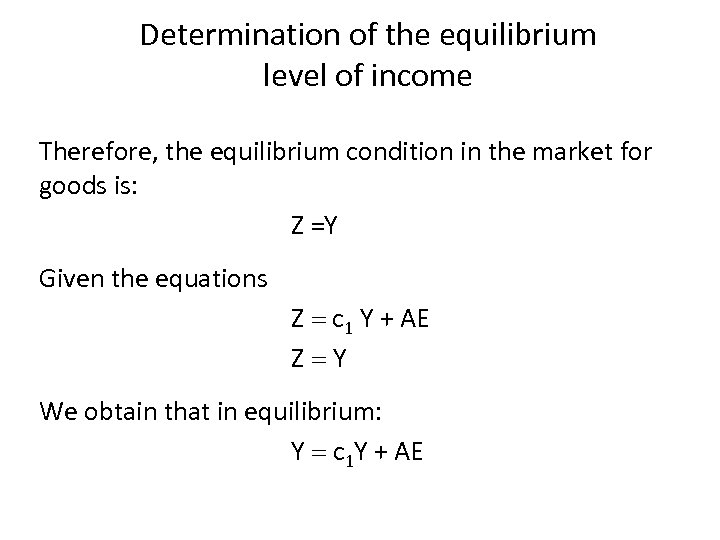Determination of the equilibrium level of income Therefore, the equilibrium condition in the market for goods is: Z =Y Given the equations Z = c 1 Y + AE Z=Y We obtain that in equilibrium: Y = c 1 Y + AE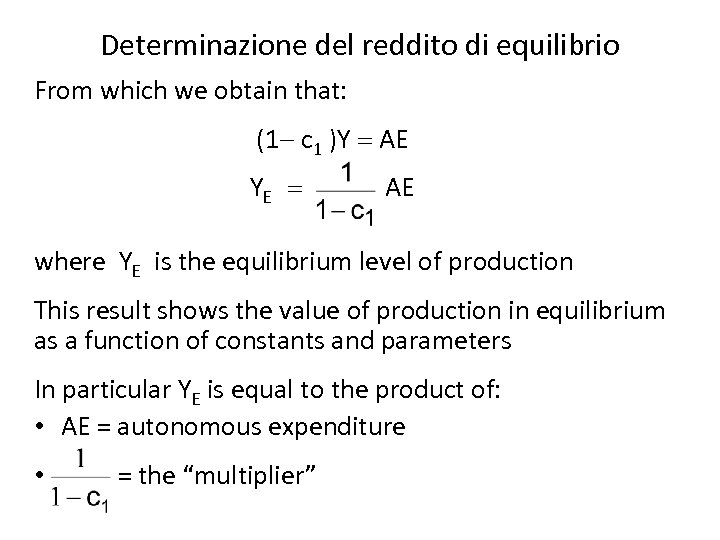Determinazione del reddito di equilibrio From which we obtain that: (1 - c 1 )Y = AE YE = AE where YE is the equilibrium level of production This result shows the value of production in equilibrium as a function of constants and parameters In particular YE is equal to the product of: • AE = autonomous expenditure • = the “multiplier”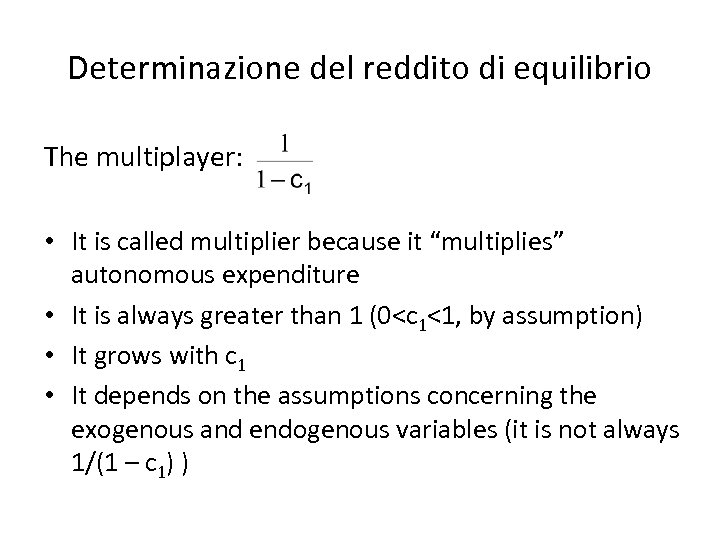Determinazione del reddito di equilibrio The multiplayer: • It is called multiplier because it “multiplies” autonomous expenditure • It is always greater than 1 (0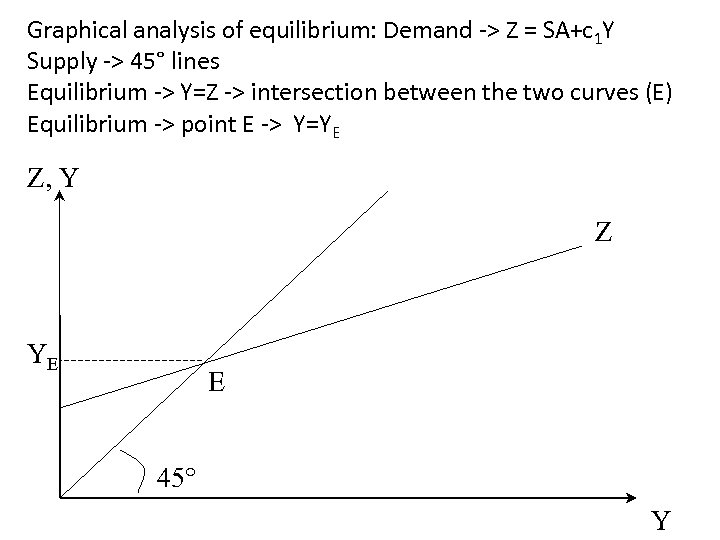Graphical analysis of equilibrium: Demand -> Z = SA+c 1 Y Supply -> 45° lines Equilibrium -> Y=Z -> intersection between the two curves (E) Equilibrium -> point E -> Y=YE Z, Y Z YE E 45° Y